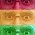## Friday, 18 January 2019

### It may be useful sometimes to use C-language library functions in your Gambas programs.

#### There may be a performance advantage in writing your own C functions or you may simply wish to use existing C libraries.

Following on from my earlier post on Gambas Libraries, this post uses simple C-code examples as a means of describing the principles of using C libraries.

I want to build an example where Gambas uses functions from 2 dynamic (shared) libraries (#1 & #2), and where library #1 also uses functions from library #2.

This is rather more complicated that using a single C library, but I hope this example will provide a better understanding of the subject.

### the C code

The first library source file (mathlib1.c) has two functions:-
• timesTwo; this just multiplies a number by 2
• timesTwoPlusNine; multiplies a number by 2 then adds 9

The second library source file (mathlib2.c) has 1 function:-
• plusNine; simply adds 9 to a number

// mathlib1.c
//trivial maths library

#include "mathlib1.h"
#include "mathlib2.h"

extern int plusNine (int anInteger);

int timesTwo (int anInteger)
{
int x;
x = 2 * anInteger;
return x;
}

int timesTwoPlusNine (int anInteger)
{
int a, b;
a = 2 * anInteger;
b = plusNine (a);
return b;
}

As you can see, the timesTwoPlusNine(int) function depends upon an EXTERNal function: plusNine(int).

//mathlib2.c
//trivial maths function

#include "mathlib1.h"
#include "mathlib2.h"

int plusNine (int anInteger)
{
int x;
x = anInteger + 9;
return x;
}

We also need header files, mathlib1.h:-

extern int timesTwo (int anInteger) ;

...and mathlib2.h:-

extern int plusNine (int anInteger) ;

Both files need to be compiled, so open a terminal at the current directory and type:-

gcc -fPIC -c mathlib1.c
gcc -fPIC -c mathlib2.c

...which produces object files; mathlib1.o & mathlib2.o

Create a shared library from mathlib2.o:-

gcc -shared -o mathlib2.so mathlib2.o

Create a shared library from mathlib1.o linking with mathlib2.o:-

gcc -shared -o mathlib1.so mathlib1.o mathlib2.o

We should end up with a shared library mathlib2.so with no dependency to mathlib1
and a shared library mathlib1.so linked to mathlib2

### Gambas test code

I started by creating a simple Gambas gui app with one ValueBox, one Button and three TextBoxes. Here are the declarations:-

Library "/home/steve/C_stuff/codeexamples/mathlib1"

Public Extern timesTwo(iNum As Integer)As Integer
Public Extern timesTwoPlusNine(iNum As Integer)As Integer

Library "/home/steve/C_stuff/codeexamples/mathlib2"

Public Extern plusNine(iNum As Integer)As Integer

Note: the Library path applies to all external functions that follow, until the next stated Library path.

The rest of the Gambas code:-

Public Sub Form_Open()
vbNumber.Value = 5
End

Public Sub Button1_Click()
TextBox1.Text = timesTwo(vbNumber.Value)
TextBox2.Text = plusNine(vbNumber.Value)
TextBox3.Text = timesTwoPlusNine(vbNumber.Value)
End

When the Gambas project is run in the IDE you should get the expected results.

### conclusion

This example should illustrate how to compile, link and create shared (dynamic) C libraries for use with Gambas. Creating multiple libraries which have inter-dependencies is clearly more complicated than just linking to a single library. But I hope this example conveys what is required for both simple and more complex configurations.

And of course you can use existing C libraries such as the C Standard Library. You just need to study the library code and any documentation

http://captainbodgit.blogspot.com/2019/01/gambas-using-external-gambas-libraries.html

http://gambaswiki.org/wiki/lang/extdecl

http://gambaswiki.org/wiki/howto/extern

1.Hi,
thanks for this great article!

I tried this and it works perfect. Now I want to use a external library (libmodbus) within the shared library for Gambas and I'm getting an error ("The program has returned the value: 127") not sure whats happening here... I then added the timesTwo() function to that shared library to see if this works and it does.

As soon as I call the initializer for the modbus RTU communication I'm getting the messag 127.

Any idea what I doing wrong?

1.Error 127 sounds like a path issue, but I'd really like to see your code.
Its not easy to post code here, so can I ask you to go over to the Gambas ONE forum (https://forum.gambas.one/) and post your code & question there. I will then have a look and see what I can suggest to help you.

2.Create a shared library with this:
gcc -shared -o mathlib2.so mathlib2.o mathlib1.o